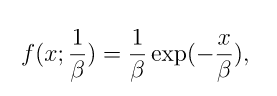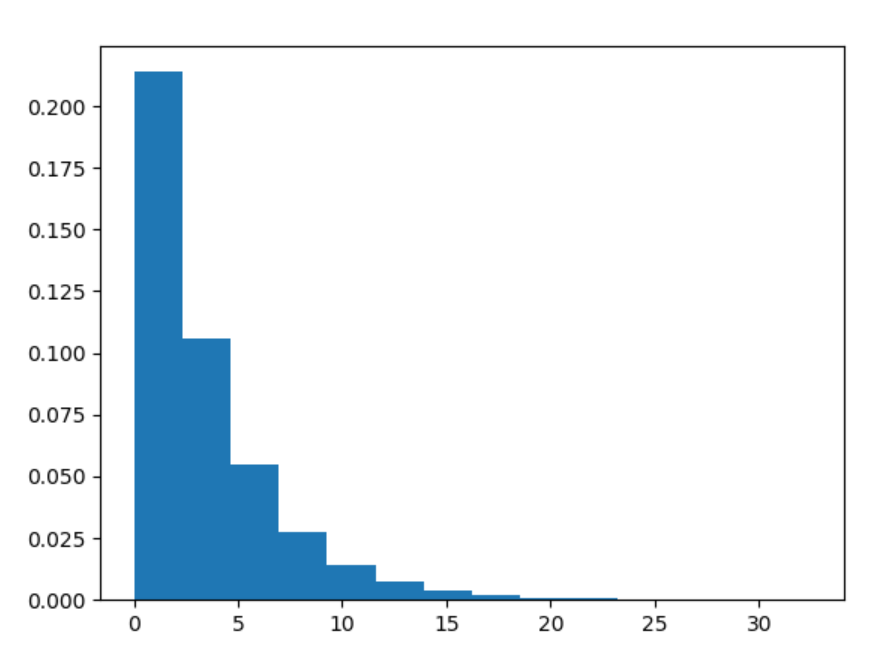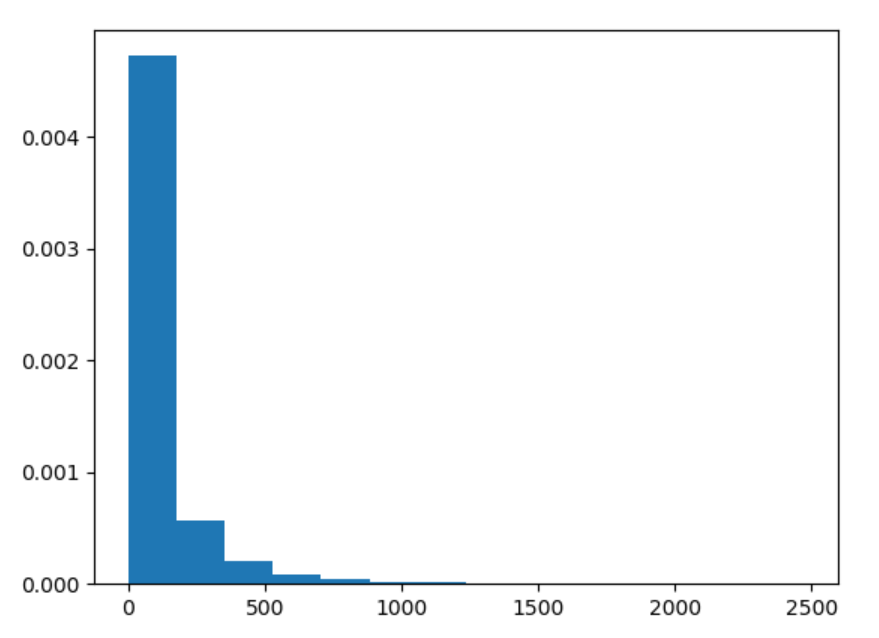Related Articles

# numpy.random.exponential() in Python

• Last Updated : 15 Jul, 2020

With the help of numpy.random.exponential() method, we can get the random samples from exponential distribution and returns the numpy array of random samples by using this method.exponential distribution

Syntax : numpy.random.exponential(scale=1.0, size=None)

Return : Return the random samples of numpy array.

Example #1 :

In this example we can see that by using numpy.random.exponential() method, we are able to get the random samples of exponential distribution and return the samples of numpy array.

## Python3

 `# import exponential``import` `numpy as np``import` `matplotlib.pyplot as plt`` ` `# Using exponential() method``gfg ``=` `np.random.exponential(``3.45``, ``10000``)`` ` `count, bins, ignored ``=` `plt.hist(gfg, ``14``, density ``=` `True``)``plt.show()`

Output :Example #2 :

## Python3

 `# import exponential``import` `numpy as np``import` `matplotlib.pyplot as plt`` ` `# Using exponential() method``gfg ``=` `np.random.exponential(``101.123``, ``10000``)``gfg1 ``=` `np.random.exponential(gfg, ``10000``)`` ` `count, bins, ignored ``=` `plt.hist(gfg1, ``14``, density ``=` `True``)``plt.show()`

Output :Attention geek! Strengthen your foundations with the Python Programming Foundation Course and learn the basics.

To begin with, your interview preparations Enhance your Data Structures concepts with the Python DS Course. And to begin with your Machine Learning Journey, join the Machine Learning – Basic Level Course

My Personal Notes arrow_drop_up